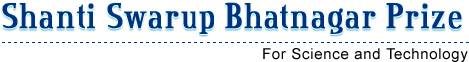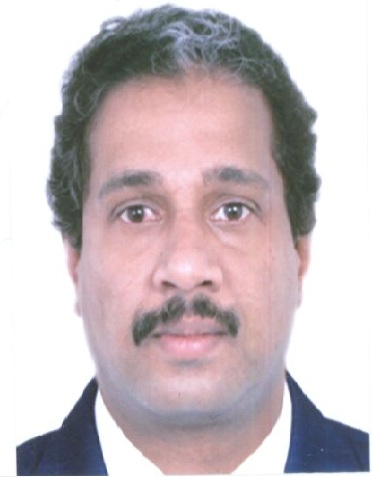• |• Home
• Brief Profile of the Awardee

## Brief Profile of the Awardee## DrK Sandeep

• 2015
• Mathematical Sciences
• 03/04/1973
• Partial Differential Equations, Variational Methods, Non-Linear Functional Analysis
##### Award Citation:

Dr Sandeep has made significant contributions to the study of partial differential equations in Hyperbolic space. He discovered the hyperbolic symmetry of solutions of the Hardy-Sobolev-Mazya equations.

 NA
 NA
 NA
 NA
##### (a) Significant contributions to science and/ or technology development by the nominee based on the work done in India during most part of last 5 years:
His work is mainly on non-compact Partial differential equations and calculus of variation. Several of the interesting problems coming from Physics and Geometry are non-compact in nature. This non-compactness reflects highly interesting phenomena related to the internal symmetries of the system which can be described in mathematical terms as separation of spheres, singularities, change in topology etc. In physical terms it indicates phase transition, particle creation etc. Sandeep has contributed significantly in the study of these type of problems. His main research contribution in the last five years has been in the study of partial differential equations in Hyperbolic space. The importance of this class of problems is that apart from the mathematical challenges it poses, it gives lots of information on several other physically relevant problems. For example, it gives a classification result for solutions of a semi-linear elliptic problem related to the Hardy-Sobolev-Maz’ya equation via hyperbolic symmetry of solutions of this equation, a fact discovered by him and his collaborators ( of 16 (b)). Hardy Sobolev Mazya type equations do come up in the study of some astrophysics models. One of his major contributions in this direction is the article  of 16 (b), where he analyses a semi-linear elliptic equation in the hyperbolic space and establishes the degeneracy/non-degeneracy of positive solutions. In this work it is established that the solutions are non-degenerate except on a finite dimensional space. The result has its consequences on the stable manifold of the Schordinger equation. In another important contribution,( of 16 (b)) he and his student has obtained multiplicity results for sign changing solutions and a nonexistence result. The highlight of the work is the sharp estimates obtained on the sign changing solutions. In an earlier work ( of 16 (a) ) he has obtained a sharp uniqueness result for positive solutions, which has consequences in the study of several other problems. This result has its implications in the study of an astrophysics model as mentioned above,where the analysis was difficult due to the lack of rotational symmetry in the problem. The work also answers a conjecture of YanYan Li and Cao regarding the locally finite energy solutions of Hardy-Sobolev-Maz’ya equations. Another striking result obtained in this article is a low dimensional phenomenon establishing nonexistence of solutions in the three dimensional hyperbolic space. This result also improves the known result which states that the best constant in the Sobolev inequality in the three dimensional hyperbolic space is the same as the corresponding one in the three dimensional Euclidean space. The work involves establishing sharp asymptotic estimates, use of classical functions like the Hypergeometric functions, moving plane method, etc. Moser-Trudinger type inequalities play an important role in the study of partial differential equations in two dimension. These inequalities are well studied in bounded domains in in the Euclidean space and also on the Euclidean sphere, but the validity of this in constant negative curvature spaces were not known.
##### (b) Impact of the contributions in the field concerned:
The results had impact on the study of uniqueness of solutions of partial differential equations coming from physics and geometry. The works developed various tools to study problems in Hyperbolic space.
 NA
 NA
 NA
 NA
FNA,FASC
 NA
 NA
 NA
 NA
 NA
 NA
 NA
 NA
##### Statement regarding collaboration with scientists abroad:
I had collaborated with a few mathematicians abroad. But most part of the work (except during my post doc period October 2002- July 2004) was done in India.
 NA
 NA

### Contact Details

• Department of Mathematics
TIFR Centre for Applicable Mathematics